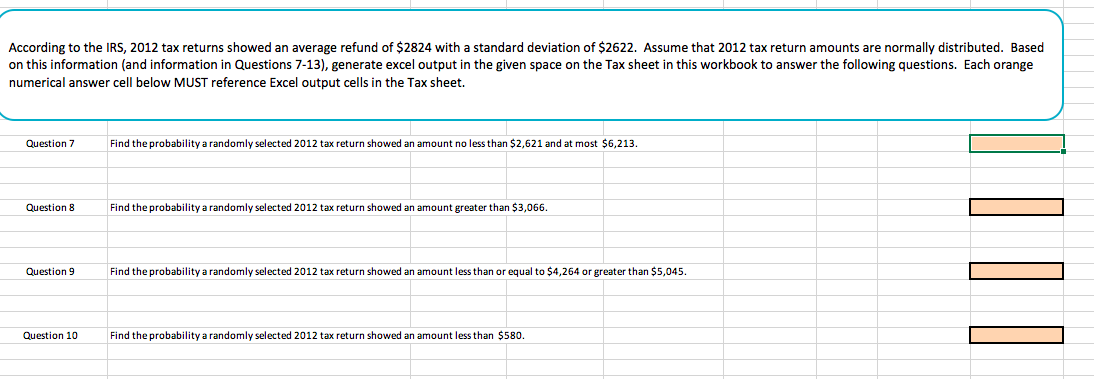# According to the IRS, 2012 tax returns showed an average refund of \$2824 with a standard deviation of \$2622. Assume that 2012 tax return amounts are normally distributed. Basedon this information (and information in Questions 7-13), generate excel output in the given space on the Tax sheet in this workbook to answer the following questions. Each orangenumerical answer cell below MUST reference Excel output cells in the Tax sheet.Question 7Find the probability a randomly selected 2012 tax return showed an amount no less than \$2,621 and at most \$6,213Question 8Find the probability a randomly selected 2012 tax return showed an amount greater than \$3,066Question 9ind the probability a randomly selected 2012 tax return showed an amount less than or equal to \$4,264 or greater than \$5,045.Question 10Find the probability a randomly selected 2012 tax return showed an amount less than \$580.

Questionhelp_outlineImage TranscriptioncloseAccording to the IRS, 2012 tax returns showed an average refund of \$2824 with a standard deviation of \$2622. Assume that 2012 tax return amounts are normally distributed. Based on this information (and information in Questions 7-13), generate excel output in the given space on the Tax sheet in this workbook to answer the following questions. Each orange numerical answer cell below MUST reference Excel output cells in the Tax sheet. Question 7 Find the probability a randomly selected 2012 tax return showed an amount no less than \$2,621 and at most \$6,213 Question 8 Find the probability a randomly selected 2012 tax return showed an amount greater than \$3,066 Question 9 ind the probability a randomly selected 2012 tax return showed an amount less than or equal to \$4,264 or greater than \$5,045. Question 10 Find the probability a randomly selected 2012 tax return showed an amount less than \$580. fullscreen
check_circleExpert Solution
Step 1

We are given that the 2012 tax returns showed an average refund of \$2824 with a standard deviation of \$2622. Also, we need to assume that 2012 tax return amounts are normally distributed.

Step 2

Question 7: Find the probability a randomly selected 2012 tax return showed an amount no less than \$2621 and at most \$6213

Answer: We can use the below excel function to find the probability a randomly selected 2012 tax return showed an amount no less than \$2621 and at most \$6213.

=NORM.DIST(6213,2824,2622,TRUE)-NORMDIST(2621,2824,2622,TRUE)=0.4328

Step 3

Question 8: Find the probability a randomly selected 2012 tax return showed an amount greater than \$3066

Answer: We can use the below excel function to find the probabilit...

### Want to see the full answer?

See Solution

#### Want to see this answer and more?

Solutions are written by subject experts who are available 24/7. Questions are typically answered within 1 hour*

See Solution
*Response times may vary by subject and question
Tagged in

### Statistics